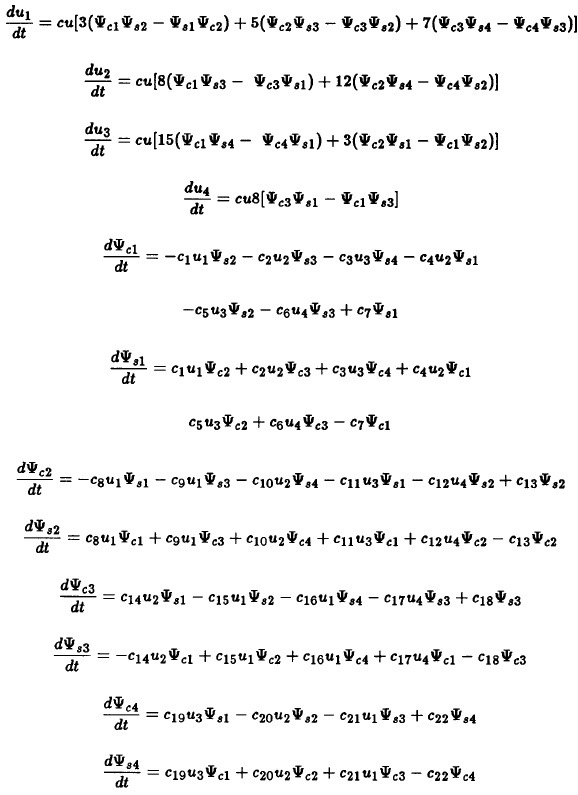APPENDIX

This appendix gives the equations for the barotropic model with 4 components to describe the zonal wind and 8 components to describe the waves. The first part gives the formula for the coefficients which do not depend on time. In these formulas k = 2π/L, where L is the length of the channel, and W is the width.With these coefficients we may write the 12 equations governing the time development of the model.From these equations it is a straightforward matter to formulate the eigen value problem by using the last 8 equations and assume that the components of the zonal wind are given. The twelve equations are used in the numerical integrations described in Section 6.